Courses

# Linear Ordinary Differential Equations of First and Second Order (Part - 1), UGC - NET Physics Physics Notes | EduRev

## Physics for IIT JAM, UGC - NET, CSIR NET

Created by: Akhilesh Thakur

## Physics : Linear Ordinary Differential Equations of First and Second Order (Part - 1), UGC - NET Physics Physics Notes | EduRev

The document Linear Ordinary Differential Equations of First and Second Order (Part - 1), UGC - NET Physics Physics Notes | EduRev is a part of the Physics Course Physics for IIT JAM, UGC - NET, CSIR NET.
All you need of Physics at this link: Physics

First Order Diﬀerential Equations

We consider the equation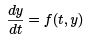Linear equations; Method of integrating factors

The function f (t, y) is a linear function in y, i.e, we can write

f (t, y) = −p(t)y + g(t).

So we will study the equation

y′ + p(t)y = g(t).                                              (A)

We introduce the method of integrating factors (due to Leibniz): We multiply equation (A) by a function µ(t) on both sides

µ(t)y′ + µ(t)p(t)y = µ(t)g(t)

The function µ is chosen such that the equation is integrable, meaning the LHS (Left Hand Side) is the derivative of something. In particular, we require:

µ(t)y′ + µ(t)p(t)y = (µ(t)y)′ , ⇒ µ(t)y′ + µ(t)p(t)y = µ(t)y′ + µ′(t)y

which requires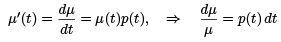Integrating both sides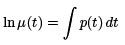which gives a formula to compute µ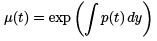Therefore, this µ is called the integrating factor.
Note that µ is not unique. In fact, adding an integration constant, we will get a diﬀerent µ. But we don’t need to be bothered, since any such µ will work. We can simply choose one that is convenient.

Putting back into equation (A), we get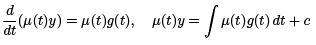which give the formula for the solution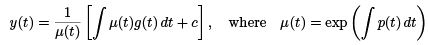Example 1. Solve y′ + ay = b (a = 0).

Answer.We have p(t) = a and g(t) = b. So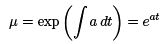so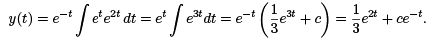where c is an arbitrary constant. Pay attention to where one adds this integration constant!

Example 2. Solve y′ + y = e2t.
We have p(t) = 1 and g(t) = e2t. So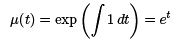and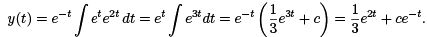Example 3. Solve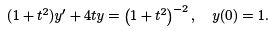Answer.First, let’s rewrite the equation into the normal form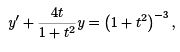so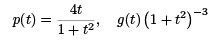Then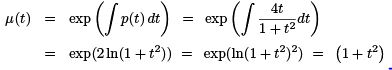Then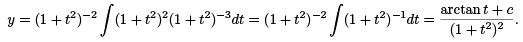By the IC y(0) = 1: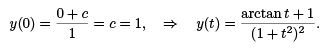Example 4. Solve ty′ − y = t2 e−t , (t > 0).
.Rewrite it into normal form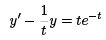so

p(t) = −1/t, g(t) = te−t.

We have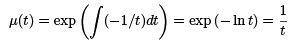and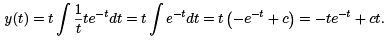Example 5. Solve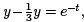with y(0) = a, and discussion the behavior of y as t → ∞, as one chooses diﬀerent initial value a.
Let’s solve it ﬁrst. We have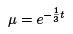so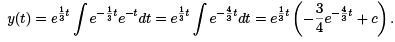Plug in the IC to ﬁnd c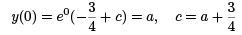so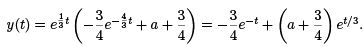To see the behavior of the solution, we see that it contains two terms. The ﬁrst term e−t goes to 0 as t grows. The second term et/3 goes to ∞ as t grows, but the constant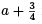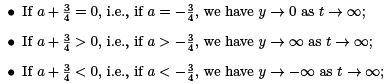On the other hand, as t → −∞, the term e−t will blow up to −∞, and will dominate.
Therefore, y → −∞ as t → −∞ for any values of a.
See plot below: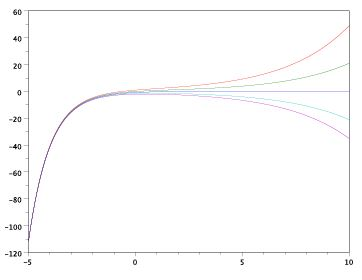Example 6. Solve ty′ + 2y = 4t2 , y(1) = 2.
Rewrite the equation ﬁrst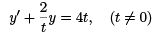So p(t) = 2/t and g(t) = 4t. We have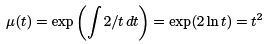and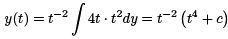By IC y (1) = 2

y(1) = 1 + c = 2, c = 1

we get the solution: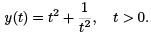Note the condition t > 0 comes from the fact that the initial condition is given at t = 1, and we require t ≠ 0.
In the graph below we plot several solutions in the t − y plan, depending on initial data.
The one for our solution is plotted with dashed line where the initial point is marked with a
‘×’.Separable Equations

We study ﬁrst order equations that can be written as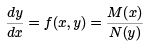where M (x) and N (y) are suitable functions of x and y only. Then we have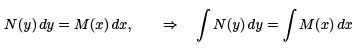and we get implicitly deﬁned solutions of y(x).
Example 1. Consider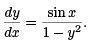We can separate the variables: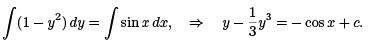If one has IC as y(π) = 2, then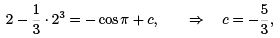so the solution y(x) is implicitly given as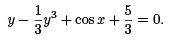Example 2. Find the solution in explicit form for the equation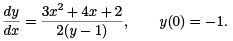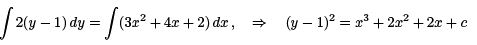Set in the IC y(0) = −1, i.e., y = −1 when x = 0, we get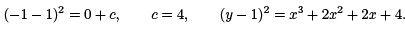In explicitly form, one has two choices: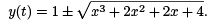To determine which sign is the correct one, we check again by the initial condition: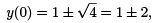must have y(0) = −1.

We see we must choose the ‘-’ sign. The solution in explicitly form is: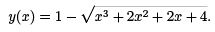On which interval will this solution be defined ?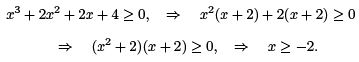We can also argue that when x = −2, we have y = 1. At this point |dy/dx| → ∞, therefore solution can not be deﬁned at this point.
The plot of the solution is given below, where the initial data is marked with ‘x’. We also include the solution with the ‘+’ sign, using dotted line.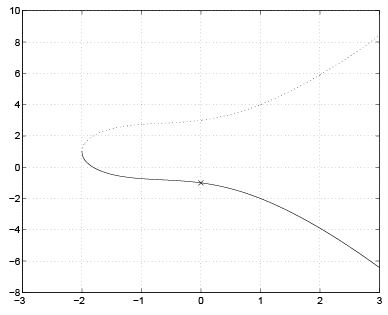Example 3. Solve y′ = 3x2 + 3x2 y2, y(0) = 0, and ﬁnd the interval where the solution is deﬁned.
Answer. Let’s ﬁrst separate the variables.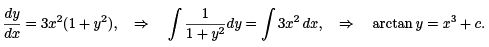Set in the IC:
arctan 0 = 0 + c, ⇒ c = 0

we get the solution

arctan y = x3 , ⇒ y = tan(x3).

Since the initial data is given at x = 0, i.e., x3 = 0, and tan is deﬁned on the interval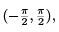We have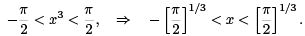Example 4. Solve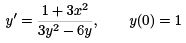and identify the interval where solution is valid.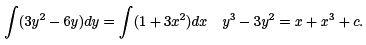Set in the IC: x = 0, y = 1, we get
1 − 3 = c, ⇒ c = −2,

Then,
y− 3y2 = x− x − 2.

Note that solution is given in implicitly form.
To ﬁnd the valid interval of this solution, we note that y′ is not deﬁned if 3y2 − 6y = 0, i.e., when y = 0 or y = 2. These are the two so-called “bad points” where you can not deﬁne the solution. To ﬁnd the corresponding values of x, we use the solution expression:

y = 0: x3+x−2 = 0,

⇒ (x+ x + 2)(x − 1) = 0, ⇒ x = 1

and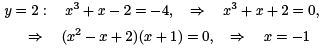(Note that we used the facts x2 + x + 2 = 0 and x2 − x + 2 = 0 for all x.) Draw the real line and work on it as following: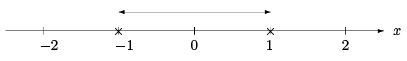Therefore the interval is −1 < x < 1.

Diﬀerences between linear and nonlinear equations

We will take this chapter before the modeling .
For a linear equation

y′ + p(t)y = g(t), y(t0) = y0,

we have the following existence and uniqueness theorem.
Theorem . If p(t) and g(t) are continuous and bounded on an open interval containing t0, then it has an unique solution on that interval.

Example 1. Find the largest interval where the solution can be deﬁned for the following problems.

(A). ty′ + y = t3, y(−1) = 3.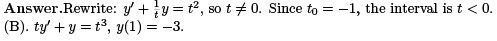Answer. The equation is same as (A), so t = 0. t0 = 1, the interval is t > 0.

(C). (t − 3)y′ + (ln t)y = 2t, y(1) = 2

Answer. Rewrite: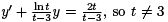and t > 0 for the ln function. Since t0 = 1, the interval is then 0 < t < 3.

(D). y′ + (tan t)y = sin t, y(π) = 100.

Answer. Since t0 = π, and for tan t to be deﬁned we must have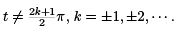So the interval is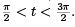For non-linear equation

y′ = f (t, y), y(t0) = y0,

we have the following theorem:

Theorem . If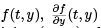are continuous and bounded on an rectangle (α < t < β , a < y < b) containing (t0, y0 ), then there exists an open interval around t0, contained in (α, β ), where the solution exists and is unique.
We note that the statement of this theorem is not as strong as the one for linear equation.
Below we give two counter examples.

Example 1. Loss of uniqueness. Consider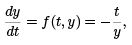y(−2) = 0.

We ﬁrst note that at y = 0, which is the initial value of y, we have y′ = f (t, y) → ∞. So the conditions of the Theorem are not satisﬁed, and we expect something to go wrong.
Solve the equation as an separable equation, we get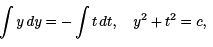and by IC we get c = (−2)2 + 0 = 4, so y2 + t2 = 4. In the y − t plan, this is the equation for a circle, centered at the origin, with radius 2. The initial condition is given at t0 = −2, y0 = 0, where the tangent line is vertical (i.e., with inﬁnite slope). We have two solutions: y =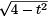and  y =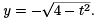We lose uniqueness of solutions.

Example 2. Blow-up of solution. Consider a simple non-linear equation:

y′ = y2, y(0) = 1.

Note that f (t, y) = y2, which is deﬁned for all t and y. But, due to the non-linearity of f , solution can not be deﬁned for all t.
This equation can be easily solved as a separable equation.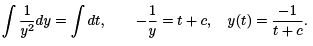By IC y(0) = 1, we get 1 = −1/(0 + c), and so c = −1, and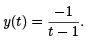We see that the solution blows up as t → 1, and can not be deﬁned beyond that point.

This kind of blow-up phenomenon is well-known for nonlinear equations.

159 docs

,

,

,

,

,

,

,

,

,

,

,

,

,

,

,

,

,

,

,

,

,

,

,

,

;